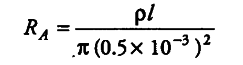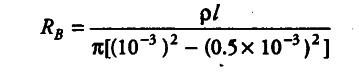# Two conductors are made of the same material

Two conductors are made of the same material and have the same length. Conductor A is a solid wire of diameter 1 mm. Conductor Sis a hollow tube of outer diameter 2 mm and inner diameter 1 mm. Find the ratio of resistance \$R_{ A }\$ to \$R_{ B }\$.

The resistance of first conductorThe resistance of second conductor,Now, the ratio of two resistors is given by